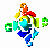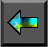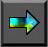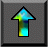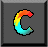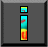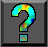Next: Chapter Notes Up: 10 Random Numbers Previous: 10.4 Summary

# Exercises

1. An application running on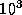processors consumes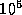random numbers per second per processor. For how long can the application execute before it exhausts all available numbers, assuming that the leapfrog method is applied to the linear congruential generator of Equation 10.3 on a 32-bit machine? A 64-bit machine?

2. A Monte Carlo simulation must perform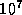independent trials. How many random numbers can be employed in each trial without duplication, assuming that the modified leapfrog method is applied to the linear congruential generator of Equation 10.1 on a 32-bit machine? A 64 bit machine?

3. Monte Carlo integration techniques are sometimes to compute integrals of high dimension. They approximate the r-dimensional integral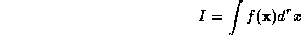of a function f as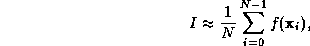where each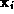is an r -vector of random values. Design and implement a parallel algorithm for this method, and use it to compute the one-dimensional integral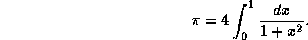Measure and account for the performance of the parallel program as a function of N and P.Next: Chapter Notes Up: 10 Random Numbers Previous: 10.4 Summary